library(tidyverse)
library(crackdownsphilanthropy)
library(rstan)
library(tidybayes)
library(broom)
library(ggstance)
library(ggdag)
library(ggraph)
library(patchwork)
library(pander)
library(scales)
library(janitor)
library(here)

source(here("analysis", "options.R"))

# qwraps2::lazyload_cache_dir("02_analysis_cache/html")

Causal pathway

Our theory and hypotheses are laid out in the causal pathway below. Our outcomes (Y: % likely to donate and amount donated) “listen to” or respond to C (crackdown), which is our main treatment or exposure. Funding (F) and issue (I) serve as heuristics for donation and influence a government’s decision to crack down on NGOs, hence the dual arrows to C and Y.

theory_dag <- dagify(Y ~ I + C + F,
C ~ I + F,
outcome = "Y",
exposure = "C") %>%
tidy_dagitty(layout = "sugiyama")

plot_dag <- ggplot(theory_dag, aes(x = x, y = y, xend = xend, yend = yend)) +
geom_dag_point(size = 6) +
geom_dag_edges(start_cap = circle(4, "mm"),
end_cap = circle(4, "mm")) +
geom_dag_text(size = pts(6), family = "Roboto Condensed", fontface = "bold") +
scale_dag() +
theme_ngos() +
theme(panel.grid = element_blank(),
axis.title = element_blank(),
axis.text = element_blank())

plot_dag %T>%
print() %T>%
ggsave(., filename = here("analysis", "output", "figures", "causal-path.pdf"),
width = 10/3, height = 2, units = "in", device = cairo_pdf) %>%
ggsave(., filename = here("analysis", "output", "figures", "causal-path.png"),
width = 10/3, height = 2, units = "in", type = "cairo", dpi = 300)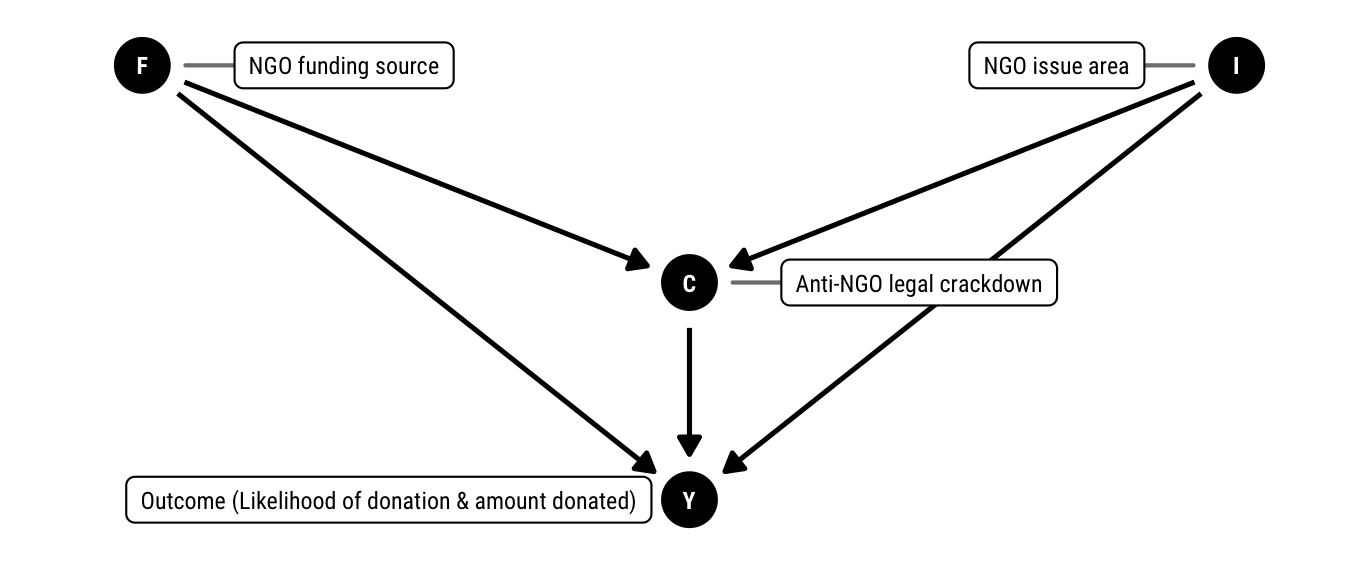Overview of data

Balance of experimental conditions

results %>% count(crackdown, issue, funding) %>%
rename(Crackdown = crackdown, Issue = issue, Funding = funding) %>%
pandoc.table() %>%
pandoc.table.return(caption = "Balance of experimental conditions {#tbl:experimental-conditions}") %>%
cat(file = here("analysis", "output", "tables", "tbl-experimental-conditions.md"))
Crackdown Issue Funding n
No crackdown Human rights Government 68
No crackdown Human rights Private 64
No crackdown Humanitarian assistance Government 68
No crackdown Humanitarian assistance Private 65
Crackdown Human rights Government 65
Crackdown Human rights Private 65
Crackdown Humanitarian assistance Government 68
Crackdown Humanitarian assistance Private 68
Total - - 531

Descriptive statistics table

vars_to_summarize <- tribble(
~variable, ~clean_name,
"donate_likely", "Likelihood of donation",
"donate_likely_bin", "Likelihood of donation (binary)",
"amount_donate", "Amount hypothetically donated ($)", "gender", "Gender", "age", "Age", "income", "Income", "education", "Education", "religiosity", "Frequency of attending religious services", "ideology", "Political views", "political_knowledge", "Frequency of following public affairs", "give_charity", "Frequency of charitable donations", "volunteer", "Volunteered in past 12 months", "favor_humanitarian", "Prior favorability towards humanitarian NGOs", "favor_humanitarian_bin", "Prior favorability towards humanitarian NGOs (binary)", "favor_human_rights", "Prior favorability towards human rights NGOs", "favor_human_rights_bin", "Prior favorability towards human rights NGOs (binary)", "favor_development", "Prior favorability towards development NGOs", "favor_development_bin", "Prior favorability towards development NGOs (binary)", "check2", "Attention check 2" ) results_summary_stats <- results %>% select(one_of(vars_to_summarize$variable)) %>%
gather(variable, value) %>%
group_by(variable) %>%
nest() %>%
mutate(N = data %>% map_int(~ nrow(.)),
summary = map2(.x = data, .y = variable, ~ md_summary_row(.x$value, .y, results))) %>% left_join(vars_to_summarize, by = "variable") %>% mutate(variable = factor(variable, levels = vars_to_summarize$variable, ordered = TRUE)) %>%
arrange(variable) %>%
select(-data, -variable) %>%
unnest(summary) %>%
select(Variable = clean_name, N,   = spark, Details = summary)

results_summary_stats %>%
select(-N) %>%
pandoc.table.return(caption = "Descriptive statistics {#tbl:descriptive-stats}",
split.cell = 80, split.table = Inf) %T>%
cat(file = here("analysis", "output", "tables", "tbl-descriptive-stats.md")) %>%
cat()
Descriptive statistics {#tbl:descriptive-stats}
Variable Details
Likelihood of donation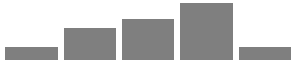Extremely unlikely (46; 8.7%) | Somewhat unlikely (110; 20.7%) | Neither likely nor unlikely (138; 26.0%) | Somewhat likely (192; 36.2%) | Extremely likely (45; 8.5%)
Likelihood of donation (binary)Not likely (294; 55.4%) | Likely (237; 44.6%)
Amount hypothetically donated ($)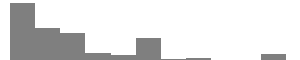Median: 10 | Mean: 22.4 | Std. Dev.: 25.67 Gender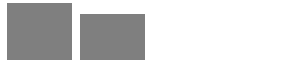Female (291; 54.8%) | Male (237; 44.6%) | Other (1; 0.2%) | Prefer not to say (2; 0.4%) Age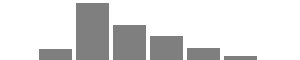Under 18 (1; 0.2%) | 18 – 24 (43; 8.1%) | 25 – 34 (207; 39.0%) | 35 – 44 (129; 24.3%) | 45 – 54 (90; 16.9%) | 55 – 64 (44; 8.3%) | 65 – 74 (16; 3.0%) | 75 – 84 (1; 0.2%) Income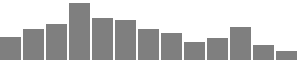Less than$10,000 (32; 6.0%) | $10,000 –$19,999 (43; 8.1%) | $20,000 –$29,999 (50; 9.4%) | $30,000 –$39,999 (78; 14.7%) | $40,000 –$49,999 (58; 10.9%) | $50,000 –$59,999 (55; 10.4%) | $60,000 –$69,999 (43; 8.1%) | $70,000 –$79,999 (38; 7.2%) | $80,000 –$89,999 (25; 4.7%) | $90,000 –$99,999 (30; 5.6%) | $100,000 –$149,999 (45; 8.5%) | More than $150,000 (21; 4.0%) | Prefer not to say (13; 2.4%) Education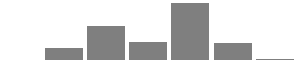Less than high school (2; 0.4%) | High school graduate (47; 8.9%) | Some college (128; 24.1%) | 2 year degree (68; 12.8%) | 4 year degree (212; 39.9%) | Graduate or professional degree (66; 12.4%) | Doctorate (8; 1.5%) Frequency of attending religious services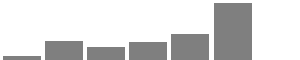More than once a week (19; 3.6%) | Once a week (74; 13.9%) | Once or twice a month (50; 9.4%) | A few times a year (71; 13.4%) | Seldom (98; 18.5%) | Never (215; 40.5%) | Don’t know (4; 0.8%) Political views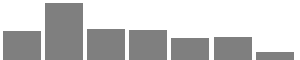Strong liberal (76; 14.3%) | Liberal (150; 28.2%) | Independent, leaning liberal (82; 15.4%) | Independent (80; 15.1%) | Independent, leaning conservative (60; 11.3%) | Conservative (61; 11.5%) | Very conservative (22; 4.1%) Frequency of following public affairs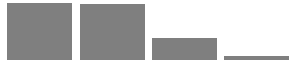Most of the time (217; 40.9%) | Some of the time (213; 40.1%) | Only now and then (84; 15.8%) | Hardly at all (17; 3.2%) Frequency of charitable donations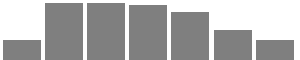Once a week (37; 7.0%) | Once a month (105; 19.8%) | Once every three months (105; 19.8%) | Once every six months (102; 19.2%) | Once a year (89; 16.8%) | Once every few years (56; 10.5%) | Never (37; 7.0%) Volunteered in past 12 months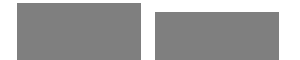No (288; 54.24%) | Yes (243; 45.76%) Prior favorability towards humanitarian NGOs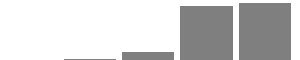Very unfavorable (2; 0.4%) | Unfavorable (6; 1.1%) | Neutral (39; 7.3%) | Favorable (235; 44.3%) | Very favorable (249; 46.9%) Prior favorability towards humanitarian NGOs (binary)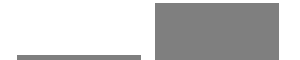Not favorable (47; 8.9%) | Favorable (484; 91.1%) Prior favorability towards human rights NGOs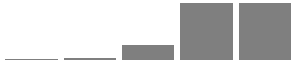Very unfavorable (5; 0.9%) | Unfavorable (12; 2.3%) | Neutral (61; 11.5%) | Favorable (226; 42.6%) | Very favorable (227; 42.7%) Prior favorability towards human rights NGOs (binary)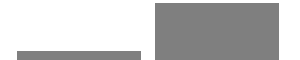Not favorable (78; 14.7%) | Favorable (453; 85.3%) Prior favorability towards development NGOs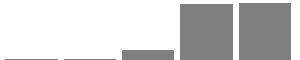Very unfavorable (5; 0.9%) | Unfavorable (8; 1.5%) | Neutral (45; 8.5%) | Favorable (235; 44.3%) | Very favorable (238; 44.8%) Prior favorability towards development NGOs (binary)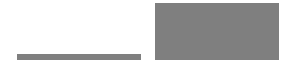Not favorable (58; 10.9%) | Favorable (473; 89.1%) Attention check 2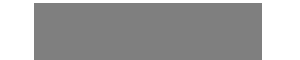Correct (531; 100%) Average likelihood and amount donated across conditions conditions_summary <- bind_rows(group_by(results, crackdown, issue, funding) %>% nest(), group_by(results, crackdown, issue) %>% nest(), group_by(results, crackdown) %>% nest(), results %>% nest()) %>% arrange(crackdown, issue, funding) %>% mutate(summary = data %>% map(~ summarize(., pct_likely = table(donate_likely_bin)[["Likely"]] / length(donate_likely_bin), mean_donation = mean(amount_donate, na.rm = TRUE), sd_donation = sd(amount_donate, na.rm = TRUE), N = nrow(.)))) %>% unnest(summary) %>% select(-data) conditions_summary_clean <- conditions_summary %>% mutate(funding = ifelse(is.na(funding) & !is.na(issue) , "*Total*", as.character(funding)), issue = ifelse(is.na(issue) & !is.na(crackdown), "*Total*", as.character(issue)), crackdown = ifelse(is.na(crackdown), "*Total*", as.character(crackdown))) %>% group_by(crackdown) %>% mutate(issue = replace(issue, duplicated(issue), NA)) %>% ungroup() %>% mutate(crackdown = replace(crackdown, duplicated(crackdown), NA)) %>% mutate(pct_likely = percent(pct_likely)) %>% rename(Crackdown condition = crackdown, Issue condition = issue, Funding condition = funding, % likely to donate = pct_likely, Amount donated (mean) = mean_donation, Amount donated (sd) = sd_donation) conditions_summary_clean %T>% pandoc.table() %>% pandoc.table.return(caption = "Average likelihood and amount donated across experimental conditions {#tbl:avg-results}") %>% cat(file = here("analysis", "output", "tables", "tbl-avg-results.md")) Crackdown condition Issue condition Funding condition % likely to donate Amount donated (mean) Amount donated (sd) N No crackdown Human rights Government 47.1% 22.4 24.8 68 Private 39.1% 19.3 22.4 64 Total 43.2% 20.9 23.6 132 Humanitarian assistance Government 44.1% 17.9 21.4 68 Private 40.0% 21.9 26.9 65 Total 42.1% 19.9 24.2 133 Total 42.6% 20.4 23.9 265 Crackdown Human rights Government 29.2% 19.4 25.8 65 Private 58.5% 28 26.4 65 Total 43.8% 23.7 26.3 130 Humanitarian assistance Government 51.5% 30.4 32.7 68 Private 47.1% 19.8 21.5 68 Total 49.3% 25.1 28.1 136 Total 46.6% 24.4 27.2 266 Total 44.6% 22.4 25.7 531 Visualize important variables Likelihood of donation donate_summary <- results %>% count(donate_likely) %>% mutate(perc = n / sum(n)) %>% mutate(highlight = ifelse(donate_likely %in% c("Extremely likely", "Somewhat likely"), TRUE, FALSE)) plot_donate_summary <- ggplot(donate_summary, aes(x = n, y = donate_likely, fill = highlight)) + geom_barh(stat = "identity") + scale_x_continuous(sec.axis = sec_axis(~ . / sum(donate_summary$n),
labels = percent_format(accuracy = 1))) +
scale_fill_manual(values = ngo_cols("green", "blue", name = FALSE), guide = FALSE) +
labs(x = NULL, y = NULL) +
theme_ngos() +
theme(panel.grid.major.y = element_blank())

plot_donate_summary %T>%
print() %T>%
ggsave(., filename = here("analysis", "output", "figures", "donate_summary.pdf"),
width = 9, height = 4, units = "in", device = cairo_pdf) %>%
ggsave(., filename = here("analysis", "output", "figures", "donate_summary.png"),
width = 9, height = 4, units = "in", type = "cairo", dpi = 300)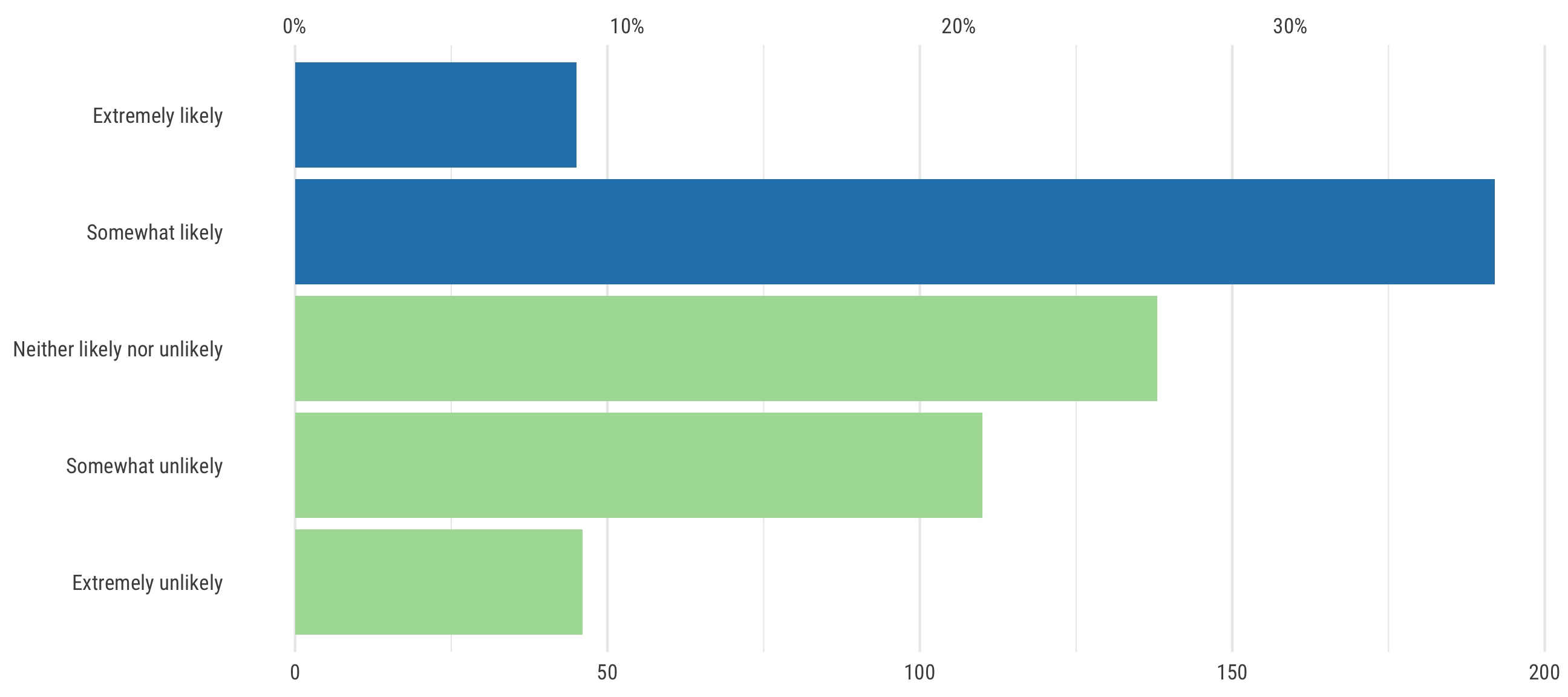Amount donated

plot_amount_summary <- ggplot(results, aes(x = amount_donate)) +
geom_histogram(bins = 20, fill = ngo_cols("blue")) +
scale_x_continuous(labels = dollar) +
labs(x = NULL, y = NULL) +
theme_ngos() +
theme(panel.grid.major.x = element_blank(),
panel.grid.minor.x = element_blank())

plot_amount_summary %T>%
print() %T>%
ggsave(., filename = here("analysis", "output", "figures", "amount_summary.pdf"),
width = 9, height = 2.75, units = "in", device = cairo_pdf) %>%
ggsave(., filename = here("analysis", "output", "figures", "amount_summary.png"),
width = 9, height = 2.75, units = "in", type = "cairo", dpi = 300)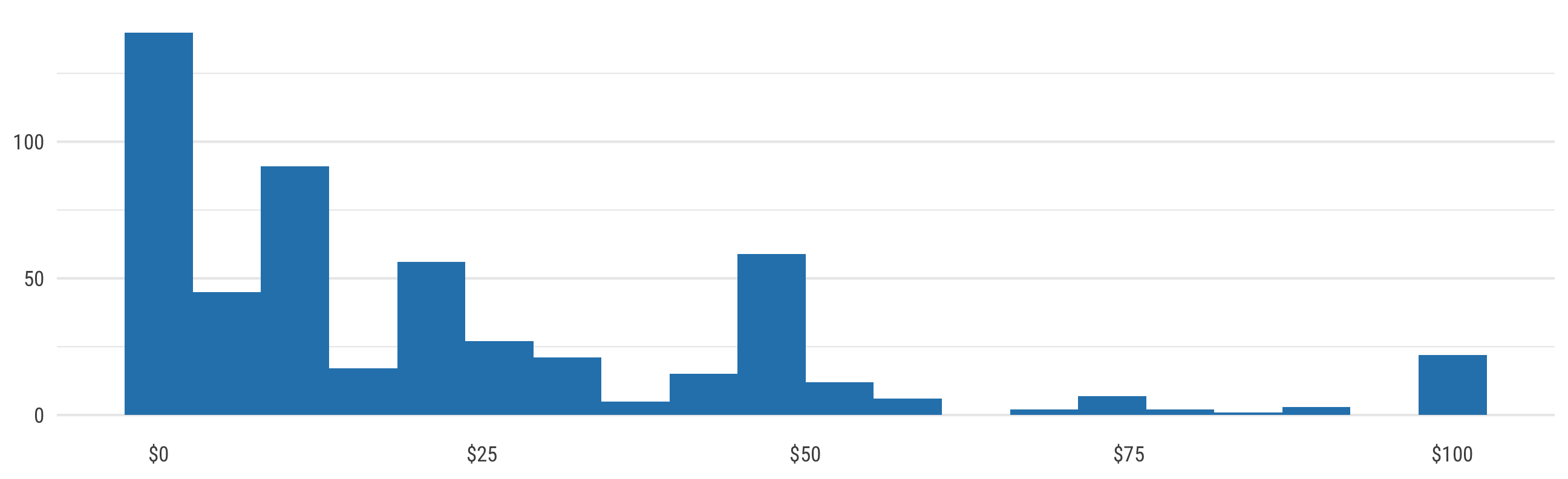all_models <- tribble(
~title, ~df,
"Crackdown", results,
# Ha, cheat here by renaming the issue and funding columns to crackdown.
# All other tests are based on the crackdown column except these two, so
# rather than build all sorts of convoluted arguments and functions, we just
# rename these as issue and funding
"Issue", mutate(results, crackdown = issue),
"Funding", mutate(results, crackdown = funding),
# Create all the nested conditions
"Human rights | Crackdown", filter(results, issue == "Human rights"),
"Humanitarian assistance | Crackdown", filter(results, issue != "Human rights"),
"Government | Crackdown", filter(results, funding == "Government"),
"Private | Crackdown", filter(results, funding == "Private"),
"Human rights | Government | Crackdown", filter(results, issue == "Human rights", funding == "Government"),
"Human rights | Private | Crackdown", filter(results, issue == "Human rights", funding == "Private"),
"Humanitarian assistance | Government | Crackdown", filter(results, issue != "Human rights", funding == "Government"),
"Humanitarian assistance | Private | Crackdown", filter(results, issue != "Human rights", funding == "Private")
) 

Treatment effects: Likelihood of donation

This time around, we’re not using interactionful regression models to calculate all these differences in groups. Instead we use real live Stan code to estimate the differences in group means and proportions!

Priors and models

We estimate the proportion of people responding that they’d be likely to donate to the organization with a binomial distribution, with a prior $$\theta$$ distribution of $$\text{Beta}(5, 5)$$. We build the following model in Stan:

\begin{aligned} n_{\text{group 1, group 2}} &\sim \text{Binomial}(n_{\text{total in group}}, \theta_{\text{group}}) &\text{[likelihood]}\\ \text{Difference} &= n_{\text{group 2}} - n_{\text{group 1}} &\text{[difference in proportions]} \\ n &: \text{Number likely to donate} \\ \\ \theta_{\text{group 1, group 2}} &\sim \text{Beta}(5, 5) &\text{[prior prob. of being likely to donate]} \end{aligned}

likely_theta <- ggplot(data = tibble(x = c(0, 1)), aes(x = x)) +
geom_area(stat = "function", fun = dbeta, args = list(shape1 = 5, shape2 = 5),
fill = "grey80", color = "black") +
labs(x = expression(Probability ~ of ~ being ~ likely ~ to ~ donate ~ (theta)), y = "Density") +
annotate(geom = "label", x = 0.5, y = 1, label = "Beta(5, 5)", size = pts(9)) +
theme_ngos(base_size = 9, density = TRUE)
likely_theta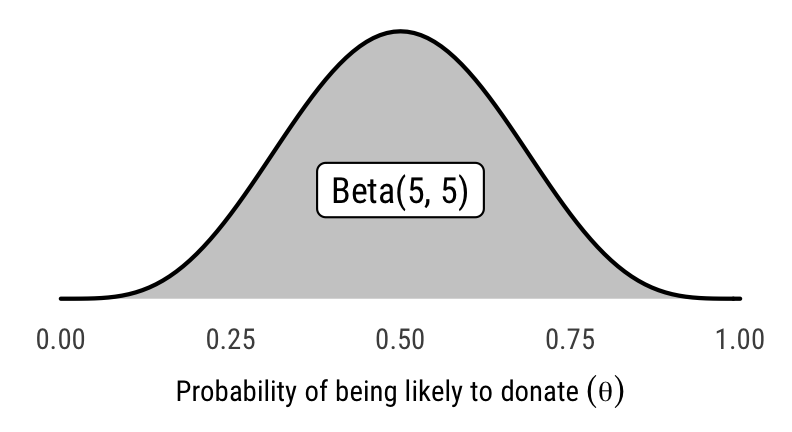likely_theta %T>%
ggsave(., filename = here("analysis", "output", "figures", "prior-likely.pdf"),
width = 2.75, height = 1.5, units = "in", device = cairo_pdf) %>%
ggsave(., filename = here("analysis", "output", "figures", "prior-likely.png"),
width = 2.75, height = 1.5, units = "in", type = "cairo", dpi = 300)

Differences

# This takes forever because of MCMC sampling, so this chunk is cached

# Sample from the compiled model
all_models_likely_run <- all_models %>%
# Simplify data for sending to Stan
mutate(stan_data_counts = df %>% map(~ {
df_counts <- .x %>%
count(crackdown, donate_likely_bin) %>%
group_by(crackdown) %>%
mutate(total = sum(n)) %>%
filter(donate_likely_bin == "Likely")

return(list(
n_total_1 = df_counts$total, n_total_2 = df_counts$total,
n_likely_1 = df_counts$n, n_likely_2 = df_counts$n
))
})) %>%
# Run the actual model on the simplified data
mutate(model = stan_data_counts %>% map(~ {
sampling(donate_likely(), data = .x, control = list(max_treedepth = 15),
chains = CHAINS, iter = ITER, warmup = WARMUP, seed = BAYES_SEED)
}))

# Extract stuff
all_models_likely <- all_models_likely_run %>%
# Extract posterior chains
mutate(posterior_chains_long = map(model, ~ {
.x %>% gather_draws(theta_1, theta_2, theta_diff, pct_change)
})) %>%
mutate(posterior_chains_wide = map(model, ~ {
.x %>% spread_draws(theta_1, theta_2, theta_diff, pct_change)
})) %>%
# Get HDI medians in tidy form
mutate(tidy = posterior_chains_long %>% map(~ {
.x %>% median_hdci() %>% to_broom_names()
}))
tidied_diffs_likely <- all_models_likely %>%
unnest(posterior_chains_long) %>%
filter(.variable == "theta_diff") %>%
mutate(category = case_when(
str_count(title, "\\|") == 0 ~ "Level 1",
str_count(title, "\\|") == 1 ~ "Level 2",
str_count(title, "\\|") == 2 ~ "Level 3"
)) %>%
mutate(title = str_remove(title, " \\| Crackdown"))

level1_likely <- tidied_diffs_likely %>%
filter(category == "Level 1") %>%
mutate(title = recode(title,
Crackdown = "Crackdown −\nNo crackdown",
Issue = "Humanitarian\nassistance −\nHuman rights",
Funding = "Private −\nGovernment\nfunding")) %>%
mutate(title = fct_inorder(title))

plot_diff_likely_a <- ggplot(level1_likely, aes(x = .value, y = fct_rev(title), fill = title)) +
geom_halfeyeh(.width = c(0.8, 0.95)) +
geom_vline(xintercept = 0) +
scale_x_continuous(labels = percent_format(accuracy = 1)) +
scale_fill_manual(values = ngo_cols(c("blue", "red", "orange"), name = FALSE), guide = FALSE) +
labs(x = "Difference in donation likelihood", y = NULL, tag = "A") +
theme_ngos(base_size = 8) +
theme(panel.grid.major.y = element_blank())

level2_likely <- tidied_diffs_likely %>%
filter(category == "Level 2") %>%
mutate(condition = case_when(
title %in% c("Human rights", "Humanitarian assistance") ~ "Issue",
title %in% c("Government", "Private") ~ "Funding"
)) %>%
mutate(facet_title = case_when(
title %in% c("Human rights", "Humanitarian assistance") ~ paste(title, "issues"),
title %in% c("Government", "Private") ~ paste(title, "funding")
)) %>%
mutate(title = case_when(
title %in% c("Humanitarian assistance", "Private") ~ "",
TRUE ~ "Crackdown −\nNo crackdown")
)

plot_diff_likely_b <- ggplot(filter(level2_likely, condition == "Issue"),
aes(x = .value, y = fct_rev(title))) +
geom_halfeyeh(.width = c(0.8, 0.95), fill = ngo_cols("red", name = FALSE)) +
geom_vline(xintercept = 0) +
scale_x_continuous(labels = percent_format(accuracy = 1)) +
labs(x = NULL, y = NULL, tag = "B") +
facet_wrap(~ facet_title, scales = "free_y") +
theme_ngos(base_size = 8) +
theme(panel.grid.major.y = element_blank())

plot_diff_likely_c <- ggplot(filter(level2_likely, condition == "Funding"),
aes(x = .value, y = fct_rev(title))) +
geom_halfeyeh(.width = c(0.8, 0.95), fill = ngo_cols("orange", name = FALSE)) +
geom_vline(xintercept = 0) +
scale_x_continuous(labels = percent_format(accuracy = 1)) +
labs(x = "Difference in donation likelihood", y = NULL, tag = "C") +
facet_wrap(~ facet_title, scales = "free_y") +
theme_ngos(base_size = 8) +
theme(panel.grid.major.y = element_blank())

level3_likely <- tidied_diffs_likely %>%
filter(category == "Level 3") %>%
separate(title, c("issue", "funding"), sep = " \\| ") %>%
mutate(issue = paste(issue, "issues"),
funding = paste(funding, "funding")) %>%
mutate(facet_title = paste0(issue, "\n", funding)) %>%
mutate(title = case_when(
funding == "Private funding" ~ "",
TRUE ~ "Crackdown −\nNo crackdown")
)

plot_diff_likely_d <- ggplot(level3_likely, aes(x = .value, y = fct_rev(title))) +
geom_halfeyeh(.width = c(0.8, 0.95), fill = ngo_cols("green", name = FALSE)) +
geom_vline(xintercept = 0) +
scale_x_continuous(labels = percent_format(accuracy = 1)) +
labs(x = "Difference in donation likelihood", y = NULL, tag = "D",
caption = "Point shows posterior median; thick black lines show 80% credible interval;\nthin black lines show 95% credible interval") +
facet_wrap(~ facet_title, scales = "free_y") +
theme_ngos(base_size = 8) +
theme(panel.grid.major.y = element_blank())

plot_diffs_likely_all <- plot_diff_likely_a /
(plot_diff_likely_b / plot_diff_likely_c) /
plot_diff_likely_d

plot_diffs_likely_all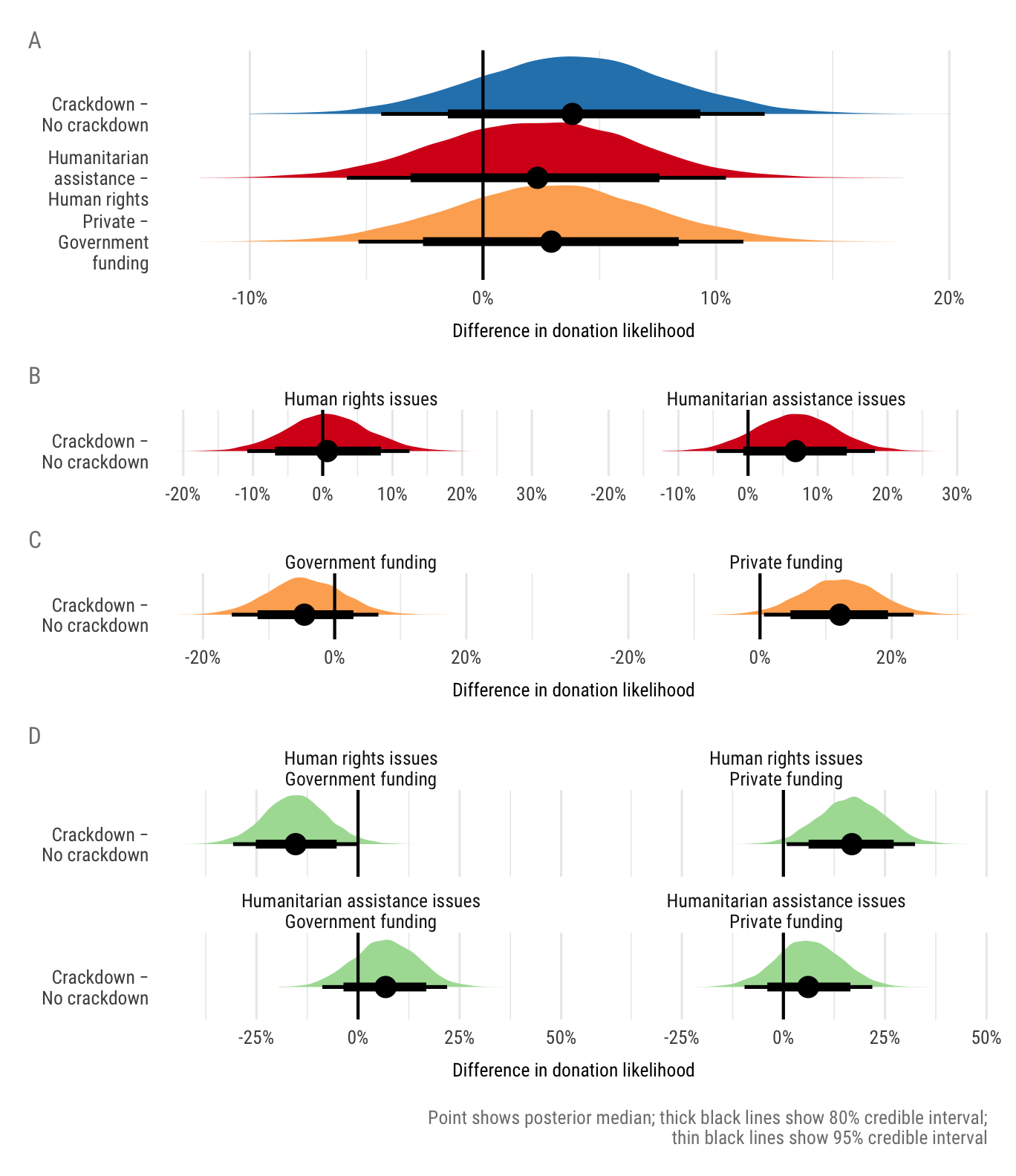plot_diffs_likely_all %T>%
ggsave(., filename = here("analysis", "output", "figures", "likely-diffs.pdf"),
width = 10/3, height = 5.5, units = "in", device = cairo_pdf) %>%
ggsave(., filename = here("analysis", "output", "figures", "likely-diffs.png"),
width = 10/3, height = 5.5, units = "in", type = "cairo", dpi = 300)
tbl_likely_tidy <- all_models_likely %>%
unnest(tidy) %>%
filter(term %in% c("theta_1", "theta_2", "theta_diff", "pct_change")) %>%
select(title, term, estimate) %>%
mutate(category = case_when(
str_count(title, "\\|") == 0 ~ "Level 1",
str_count(title, "\\|") == 1 ~ "Level 2",
str_count(title, "\\|") == 2 ~ "Level 3"
))

tbl_likely_probs <- all_models_likely %>%
unnest(posterior_chains_long) %>%
filter(.variable == "theta_diff") %>%
group_by(title) %>%
summarize(p.greater0 = mean(.value > 0),
p.less0 = mean(.value < 0),
p.diff.not0 = ifelse(median(.value) > 0, p.greater0, p.less0)) %>%
ungroup()

# Save combined table for later use in manuscript
tbl_likely_tidy %>%
left_join(tbl_likely_probs, by = "title") %>%
saveRDS(here("data", "derived_data", "results_models_likely.rds"))

tbl_likely_1 <- tbl_likely_tidy %>%
left_join(tbl_likely_probs, by = "title") %>%
filter(category == "Level 1") %>%
mutate(title = recode(title,
Crackdown = "Crackdown − No crackdown",
Issue = "*Humanitarian assistance − Human rights*",
Funding = "*Private − Government funding*")) %>%
mutate_at(vars(theta_1, theta_2, theta_diff, pct_change),
funs(percent_format(accuracy = 0.1)(.))) %>%
mutate(p.diff.not0 = as.character(round(p.diff.not0, 2))) %>%
select(Frame = title, % likely~Treatment~ = theta_2, % likely~Control~ = theta_1,
$\\Delta$ = theta_diff, $\\%\\Delta$ = pct_change,
$p(\\Delta \\neq 0)$ = p.diff.not0)
tbl_likely_2 <- tbl_likely_tidy %>%
left_join(tbl_likely_probs, by = "title") %>%
filter(category == "Level 2") %>%
mutate(title_clean = str_remove(title, " \\| Crackdown")) %>%
mutate(title_clean = case_when(
title_clean %in% c("Human rights", "Humanitarian assistance") ~ paste(title_clean, "issues"),
title_clean %in% c("Government", "Private") ~ paste(title_clean, "funding")
)) %>%
mutate(title_clean = factor(title_clean,
levels = c("Human rights issues",
"Humanitarian assistance issues",
"Government funding", "Private funding"))) %>%
arrange(title_clean) %>%
mutate_at(vars(theta_1, theta_2, theta_diff, pct_change),
funs(percent_format(accuracy = 0.1)(.))) %>%
mutate(p.diff.not0 = as.character(round(p.diff.not0, 2))) %>%
mutate(title_clean = as.character(title_clean)) %>%
select(H~2a~ and H~3a~ = title_clean,
% likely~Crackdown~ = theta_2, % likely~No\\ crackdown~ = theta_1,
$\\Delta$ = theta_diff, $\\%\\Delta$ = pct_change,
$p(\\Delta \\neq 0)$ = p.diff.not0)
tbl_likely_3 <- tbl_likely_tidy %>%
left_join(tbl_likely_probs, by = "title") %>%
filter(category == "Level 3") %>%
mutate(title_clean = str_remove(title, " \\| Crackdown")) %>%
separate(title_clean, c("Issue", "Funding"), sep = " \\| ") %>%
mutate(Issue = factor(Issue, levels = c("Human rights", "Humanitarian assistance")),
Funding = factor(Funding, levels = c("Government", "Private"))) %>%
arrange(Issue, Funding) %>%
mutate_at(vars(theta_1, theta_2, theta_diff, pct_change),
funs(percent_format(accuracy = 0.1)(.))) %>%
mutate(p.diff.not0 = as.character(round(p.diff.not0, 2))) %>%
mutate(Frame = paste0(Issue, " issues, ", Funding, " funding")) %>%
select(H~2a~ and H~3a~ (nested) = Frame,
% likely~Crackdown~ = theta_2, % likely~No\\ crackdown~ = theta_1,
$\\Delta$ = theta_diff, $\\%\\Delta$ = pct_change,
$p(\\Delta \\neq 0)$ = p.diff.not0)
tbl_likely_2_header <- enframe(colnames(tbl_likely_2)) %>%
mutate(value = (value)) %>%
set_names(colnames(tbl_likely_1))

mutate(value = (value)) %>%
set_names(colnames(tbl_likely_1))

bind_rows(tbl_likely_1,
set_names(tbl_likely_2, colnames(tbl_likely_1)),
set_names(tbl_likely_3, colnames(tbl_likely_1))) %>%
rename(H~1a~ = Frame) %>%
pandoc.table.return(caption = 'Likelihood of donation and differences in proportions in "crackdown" (treatment) and "no crackdown" (control) conditions; values represent posterior medians {#tbl:likely-diffs}',
justify = "lccccc") %T>%
cat() %>%
cat(file = here("analysis", "output", "tables", "tbl-likely-diffs.md"))
Likelihood of donation and differences in proportions in “crackdown” (treatment) and “no crackdown” (control) conditions; values represent posterior medians {#tbl:likely-diffs}
H1a % likelyTreatment % likelyControl $$\Delta$$ $$\%\Delta$$ $$p(\Delta \neq 0)$$
Crackdown − No crackdown 46.8% 42.8% 3.9% 9.2% 0.83
Private − Government funding 46.4% 43.3% 3.1% 7.1% 0.76
Humanitarian assistance − Human rights 45.9% 43.8% 2.1% 4.7% 0.7
H2a and H3a % likelyCrackdown % likelyNo crackdown $$\Delta$$ $$\%\Delta$$ $$p(\Delta \neq 0)$$
Human rights issues 44.2% 43.7% 0.6% 1.5% 0.54
Humanitarian assistance issues 49.4% 42.7% 6.8% 15.9% 0.88
Government funding 41.3% 45.9% -4.6% -10.1% 0.79
Private funding 52.5% 40.2% 12.2% 30.3% 0.98
H2a and H3a (nested) % likelyCrackdown % likelyNo crackdown $$\Delta$$ $$\%\Delta$$ $$p(\Delta \neq 0)$$
Human rights issues, Government funding 31.9% 47.5% -15.4% -32.6% 0.98
Human rights issues, Private funding 57.5% 40.5% 17.0% 41.7% 0.98
Humanitarian assistance issues, Government funding 51.2% 44.8% 6.4% 14.4% 0.8
Humanitarian assistance issues, Private funding 47.5% 41.2% 6.2% 15.2% 0.79

Treatment effects: Amount donated

Priors and models

Following John Kruschke’s “Bayesian Estimation Supersedes the t-test (BEST)” procedure, we estimate means for each group with a t-distribution. We use the following priors for the distribution parameters:

\begin{aligned} x_{\text{group 1, group 2}} &\sim \text{Student } t(\nu, \mu, \sigma) &\text{[likelihood]}\\ \text{Difference} &= x_{\text{group 2}} - x_{\text{group 1}} &\text{[difference in means]} \\ x &: \text{Mean amount donated} \\ \\ \nu &\sim \text{Exponential}(1 / 29) &\text{[prior normality]} \\ \mu_{\text{group 1, group 2}} &\sim \mathcal{N}(\bar{x}_{\text{group 1, group 2}}, 10) &\text{[prior donation mean per group]}\\ \sigma_{\text{group 1, group 2}} &\sim \text{Cauchy}(0, 1)&\text{[prior donation sd per group]} \end{aligned}

amount_nu <- ggplot(data = tibble(x = c(0, 200)), aes(x = x)) +
geom_area(stat = "function", fun = dexp, args = list(rate = 1/29),
fill = "grey80", color = "black") +
labs(x = expression(Normality ~ parameter ~ (nu)), y = "Density") +
annotate(geom = "label", x = 100, y = 0.009,
label = "Exponential(1/29)", size = pts(9)) +
theme_ngos(base_size = 9, density = TRUE)

amount_mu <- ggplot(data = tibble(x = c(0, 100)), aes(x = x)) +
geom_area(stat = "function", fun = dnorm, args = list(mean = 50, sd = 10),
fill = "grey80", color = "black") +
scale_x_continuous(breaks = c(seq(0, 100, 25)),
labels = c("−$50", "−$25", "Group average", "+$25", "+$50")) +
annotate(geom = "label", x = 50, y = 0.01, label = "N(bar(x), 10)",
parse = TRUE, size = pts(9)) +
labs(x = expression(Average ~ donated ~ (mu)), y = NULL) +
theme_ngos(base_size = 9, density = TRUE)

amount_sigma <- ggplot(data = tibble(x = c(0, 10)), aes(x = x)) +
geom_area(stat = "function", fun = dcauchy, args = list(location = 0, scale = 1),
fill = "grey80", color = "black") +
scale_x_continuous(labels = dollar) +
annotate(geom = "label", x = 5, y = 0.08, label = "Cauchy(0, 1)", size = pts(9)) +
labs(x = expression(SD ~ donated ~ (sigma)), y = NULL) +
theme_ngos(base_size = 9, density = TRUE)

amount_priors <- amount_nu + amount_mu + amount_sigma
amount_priors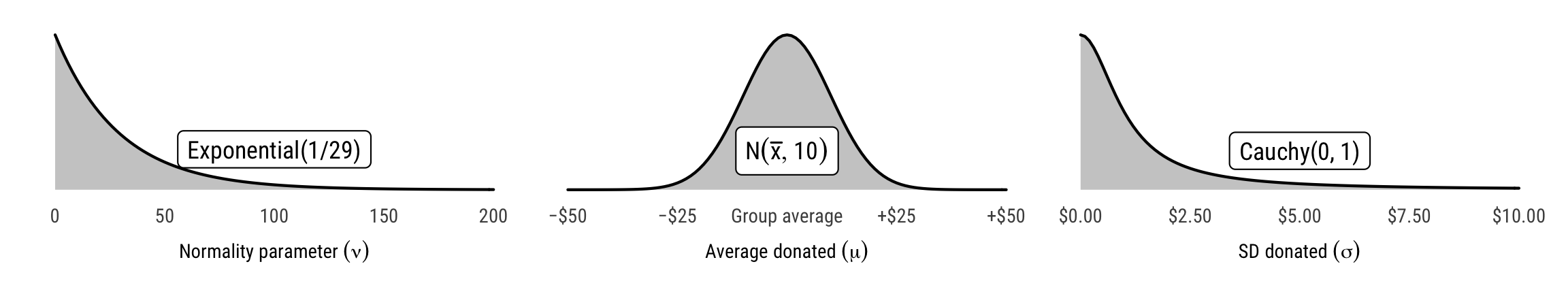amount_priors %T>%
ggsave(., filename = here("analysis", "output", "figures", "prior-amount.pdf"),
width = 8, height = 1.5, units = "in", device = cairo_pdf) %>%
ggsave(., filename = here("analysis", "output", "figures", "prior-amount.png"),
width = 8, height = 1.5, units = "in", type = "cairo", dpi = 300)

Differences

# Sample from the compiled model
all_models_amount_run <- all_models %>%
# Simplify data for sending to Stan
mutate(stan_data = df %>% map(~ {
select(.x, amount = amount_donate, group = crackdown)
})) %>%
# Run the actual model on the simplified data
mutate(model = stan_data %>% map(~ {
sampling(amount_donated_best(), data = compose_data(.x),
chains = CHAINS, iter = ITER, warmup = WARMUP, seed = BAYES_SEED)
}))

# Extract stuff
all_models_amount <- all_models_amount_run %>%
# Extract posterior chains
mutate(posterior_chains_long = map2(model, stan_data, ~ {
.x %>%
recover_types(.y) %>%
gather_draws(mu[group], sigma[group], mu_diff, pct_change, cohen_d, cles, nu, log10nu)
})) %>%
mutate(posterior_chains_wide = map2(model, stan_data, ~ {
.x %>%
recover_types(.y) %>%
spread_draws(mu[group], sigma[group], mu_diff, pct_change, cohen_d, cles, nu, log10nu)
})) %>%
# Get HDI medians in tidy form
mutate(tidy = posterior_chains_long %>% map(~ {
.x %>% median_hdci() %>% to_broom_names()
}))
# This will generate a warning because unnest() puts the crackdown values into
# one column and we cheated by renaming issue and funding as crackdown, so it
# gets mad when combining the factors in crackdown with those in issue and
# funding. But because we only care about the diffs, we don't actually need the
# category labels here, so it's all good.
tidied_diffs_amount <- all_models_amount %>%
unnest(posterior_chains_long) %>%
filter(.variable == "mu_diff") %>%
mutate(category = case_when(
str_count(title, "\\|") == 0 ~ "Level 1",
str_count(title, "\\|") == 1 ~ "Level 2",
str_count(title, "\\|") == 2 ~ "Level 3"
)) %>%
mutate(title = str_remove(title, " \\| Crackdown"))

level1_amount <- tidied_diffs_amount %>%
filter(category == "Level 1") %>%
mutate(title = recode(title,
Crackdown = "Crackdown −\nNo crackdown",
Issue = "Humanitarian\nassistance −\nHuman rights",
Funding = "Private −\nGovernment\nfunding")) %>%
mutate(title = fct_inorder(title))

plot_diff_amount_a <- ggplot(level1_amount, aes(x = .value, y = fct_rev(title), fill = title)) +
geom_vline(xintercept = 0) +
geom_halfeyeh(.width = c(0.8, 0.95)) +
scale_x_continuous(labels = dollar) +
scale_fill_manual(values = ngo_cols(c("blue", "red", "orange"), name = FALSE), guide = FALSE) +
labs(x = "Difference in amount donated", y = NULL, tag = "A") +
theme_ngos(base_size = 8) +
theme(panel.grid.major.y = element_blank())

level2_amount <- tidied_diffs_amount %>%
filter(category == "Level 2") %>%
mutate(condition = case_when(
title %in% c("Human rights", "Humanitarian assistance") ~ "Issue",
title %in% c("Government", "Private") ~ "Funding"
)) %>%
mutate(facet_title = case_when(
title %in% c("Human rights", "Humanitarian assistance") ~ paste(title, "issues"),
title %in% c("Government", "Private") ~ paste(title, "funding")
)) %>%
mutate(title = case_when(
title %in% c("Humanitarian assistance", "Private") ~ "",
TRUE ~ "Crackdown −\nNo crackdown")
)

plot_diff_amount_b <- ggplot(filter(level2_amount, condition == "Issue"),
aes(x = .value, y = fct_rev(title))) +
geom_halfeyeh(.width = c(0.8, 0.95), fill = ngo_cols("red", name = FALSE)) +
geom_vline(xintercept = 0) +
scale_x_continuous(labels = dollar) +
labs(x = NULL, y = NULL, tag = "B") +
facet_wrap(~ facet_title, scales = "free_y") +
theme_ngos(base_size = 8) +
theme(panel.grid.major.y = element_blank())

plot_diff_amount_c <- ggplot(filter(level2_amount, condition == "Funding"),
aes(x = .value, y = fct_rev(title))) +
geom_halfeyeh(.width = c(0.8, 0.95), fill = ngo_cols("orange", name = FALSE)) +
geom_vline(xintercept = 0) +
scale_x_continuous(labels = dollar) +
labs(x = "Difference in amount donated", y = NULL, tag = "C") +
facet_wrap(~ facet_title, scales = "free_y") +
theme_ngos(base_size = 8) +
theme(panel.grid.major.y = element_blank())

level3_amount <- tidied_diffs_amount %>%
filter(category == "Level 3") %>%
separate(title, c("issue", "funding"), sep = " \\| ") %>%
mutate(issue = paste(issue, "issues"),
funding = paste(funding, "funding")) %>%
mutate(facet_title = paste0(issue, "\n", funding)) %>%
mutate(title = case_when(
funding == "Private funding" ~ "",
TRUE ~ "Crackdown −\nNo crackdown")
)

plot_diff_amount_d <- ggplot(level3_amount, aes(x = .value, y = fct_rev(title))) +
geom_halfeyeh(.width = c(0.8, 0.95), fill = ngo_cols("green", name = FALSE)) +
geom_vline(xintercept = 0) +
scale_x_continuous(labels = dollar) +
labs(x = "Difference in amount donated", y = NULL, tag = "D",
caption = "Point shows posterior median; thick black lines show 80% credible interval;\nthin black lines show 95% credible interval") +
facet_wrap(~ facet_title, scales = "free_y") +
theme_ngos(base_size = 8) +
theme(panel.grid.major.y = element_blank())

plot_diffs_amounts_all <- plot_diff_amount_a /
(plot_diff_amount_b / plot_diff_amount_c) /
plot_diff_amount_d

plot_diffs_amounts_all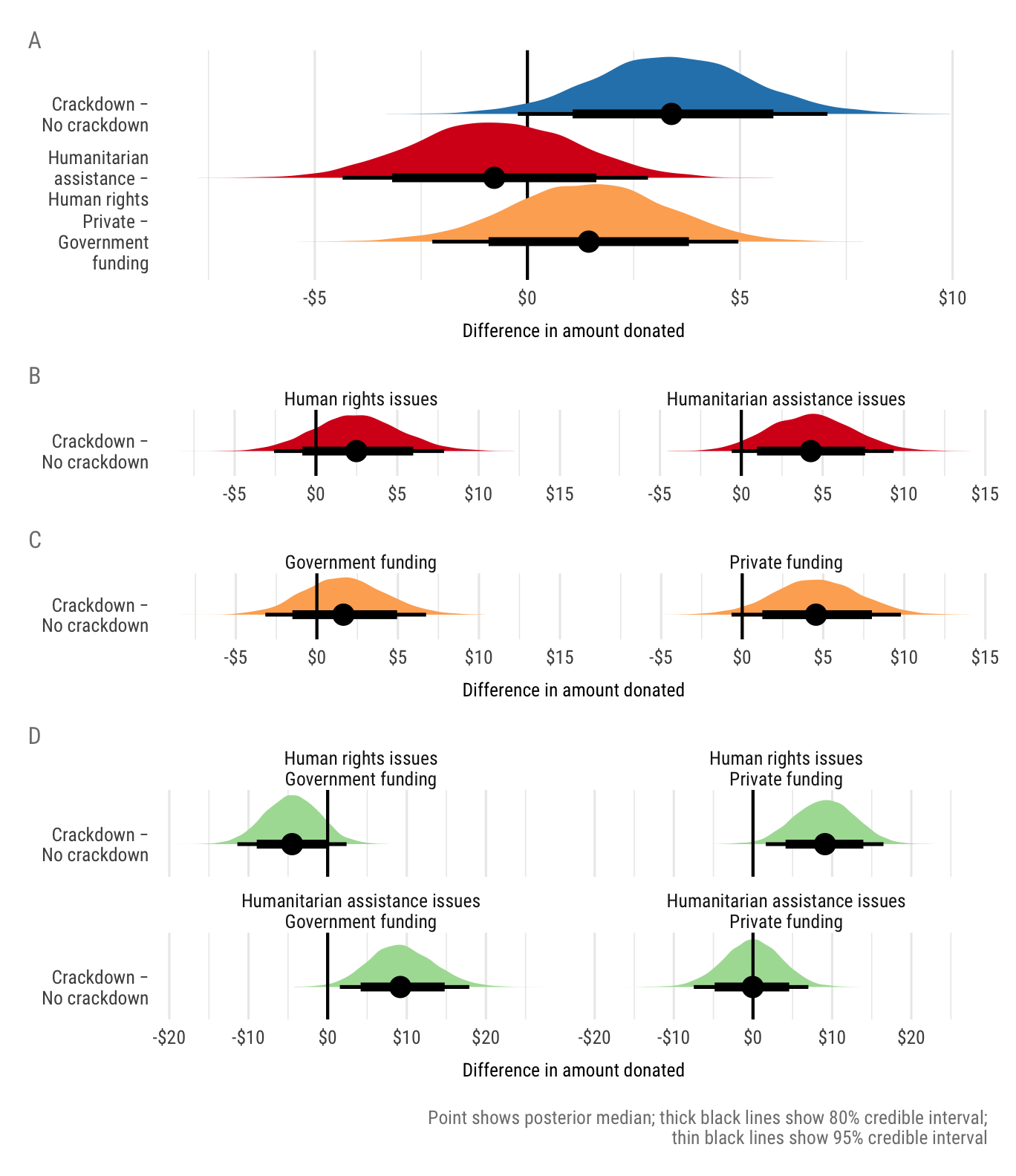plot_diffs_amounts_all %T>%
ggsave(., filename = here("analysis", "output", "figures", "amount-diffs.pdf"),
width = 10/3, height = 5.5, units = "in", device = cairo_pdf) %>%
ggsave(., filename = here("analysis", "output", "figures", "amount-diffs.png"),
width = 10/3, height = 5.5, units = "in", type = "cairo", dpi = 300)
tbl_amounts_tidy <- all_models_amount %>%
unnest(tidy) %>%
filter(term %in% c("mu", "mu_diff", "pct_change")) %>%
group_by(title) %>%
mutate(group_id = 1:n()) %>%
mutate(term = case_when(
term == "mu" ~ paste0(term, "_", group_id),
TRUE ~ term
)) %>%
select(title, term, estimate) %>%
mutate(category = case_when(
str_count(title, "\\|") == 0 ~ "Level 1",
str_count(title, "\\|") == 1 ~ "Level 2",
str_count(title, "\\|") == 2 ~ "Level 3"
)) %>%
ungroup()

tbl_amounts_probs <- all_models_amount %>%
unnest(posterior_chains_long) %>%
filter(.variable == "mu_diff") %>%
group_by(title) %>%
summarize(p.greater0 = mean(.value > 0),
p.less0 = mean(.value < 0),
p.diff.not0 = ifelse(median(.value) > 0, p.greater0, p.less0)) %>%
ungroup()

# Save combined table for later use in manuscript
tbl_amounts_tidy %>%
left_join(tbl_amounts_probs, by = "title") %>%
saveRDS(here("data", "derived_data", "results_models_amount.rds"))

tbl_amount_1 <- tbl_amounts_tidy %>%
left_join(tbl_amounts_probs, by = "title") %>%
filter(category == "Level 1") %>%
mutate(title = recode(title,
Crackdown = "Crackdown − No crackdown",
Issue = "*Humanitarian assistance − Human rights*",
Funding = "*Private − Government funding*")) %>%
mutate_at(vars(mu_1, mu_2, mu_diff, p.diff.not0), funs(as.character(round(., 2)))) %>%
mutate(pct_change = percent_format(accuracy = 0.1)(pct_change)) %>%
select(Frame = title, Amount~Treatment~ = mu_2, Amount~Control~ = mu_1,
$\\Delta$ = mu_diff, $\\%\\Delta$ = pct_change,
$p(\\Delta \\neq 0)$ = p.diff.not0)
tbl_amount_2 <- tbl_amounts_tidy %>%
left_join(tbl_amounts_probs, by = "title") %>%
filter(category == "Level 2") %>%
mutate(title_clean = str_remove(title, " \\| Crackdown")) %>%
mutate(title_clean = case_when(
title_clean %in% c("Human rights", "Humanitarian assistance") ~ paste(title_clean, "issues"),
title_clean %in% c("Government", "Private") ~ paste(title_clean, "funding")
)) %>%
mutate(title_clean = factor(title_clean,
levels = c("Human rights issues",
"Humanitarian assistance issues",
"Government funding", "Private funding"))) %>%
arrange(title_clean) %>%
mutate_at(vars(mu_1, mu_2, mu_diff, p.diff.not0), funs(as.character(round(., 2)))) %>%
mutate(pct_change = percent_format(accuracy = 0.1)(pct_change)) %>%
mutate(title_clean = as.character(title_clean)) %>%
select(H~2b~ and H~3b~ = title_clean, Amount~Crackdown~ = mu_2, Amount~No\\ crackdown~ = mu_1,
$\\Delta$ = mu_diff, $\\%\\Delta$ = pct_change,
$p(\\Delta \\neq 0)$ = p.diff.not0)
tbl_amount_3 <- tbl_amounts_tidy %>%
left_join(tbl_amounts_probs, by = "title") %>%
filter(category == "Level 3") %>%
mutate(title_clean = str_remove(title, " \\| Crackdown")) %>%
separate(title_clean, c("Issue", "Funding"), sep = " \\| ") %>%
mutate(Issue = factor(Issue, levels = c("Human rights", "Humanitarian assistance")),
Funding = factor(Funding, levels = c("Government", "Private"))) %>%
arrange(Issue, Funding) %>%
mutate(Frame = paste0(Issue, " issues, ", Funding, " funding")) %>%
mutate_at(vars(mu_1, mu_2, mu_diff, p.diff.not0), funs(as.character(round(., 2)))) %>%
mutate(pct_change = percent_format(accuracy = 0.1)(pct_change)) %>%
select(H~2b~ and H~3b~ (nested) = Frame,
Amount~Crackdown~ = mu_2, Amount~No\\ crackdown~ = mu_1,
$\\Delta$ = mu_diff, $\\%\\Delta$ = pct_change,
$p(\\Delta \\neq 0)$ = p.diff.not0)
tbl_amount_2_header <- enframe(colnames(tbl_amount_2)) %>%
mutate(value = (value)) %>%
set_names(colnames(tbl_amount_1))

mutate(value = (value)) %>%
set_names(colnames(tbl_amount_1))

bind_rows(tbl_amount_1,
set_names(tbl_amount_2, colnames(tbl_amount_1)),
set_names(tbl_amount_3, colnames(tbl_amount_1))) %>%
rename(H~1b~ = Frame) %>%
pandoc.table.return(caption = 'Mean values and differences in means for amount donated in "crackdown" (treatment) and "no crackdown" (control) conditions; values represent posterior medians {#tbl:amount-diffs}',
justify = "lccccc") %T>%
cat() %>%
cat(file = here("analysis", "output", "tables", "tbl-amount-diffs.md"))
Mean values and differences in means for amount donated in “crackdown” (treatment) and “no crackdown” (control) conditions; values represent posterior medians {#tbl:amount-diffs}
H1b AmountTreatment AmountControl $$\Delta$$ $$\%\Delta$$ $$p(\Delta \neq 0)$$
Crackdown − No crackdown 16.34 12.93 3.39 26.3% 0.97
Private − Government funding 15.13 13.71 1.42 10.4% 0.79
Humanitarian assistance − Human rights 14.06 14.85 -0.82 -5.5% 0.67
H2b and H3b AmountCrackdown AmountNo crackdown $$\Delta$$ $$\%\Delta$$ $$p(\Delta \neq 0)$$
Human rights issues 17.4 14.86 2.54 17.2% 0.83
Humanitarian assistance issues 15.91 11.68 4.3 36.9% 0.95
Government funding 13.83 12.24 1.61 13.1% 0.74
Private funding 18.95 14.23 4.62 32.4% 0.97
H2b and H3b (nested) AmountCrackdown AmountNo crackdown $$\Delta$$ $$\%\Delta$$ $$p(\Delta \neq 0)$$
Human rights issues, Government funding 10.56 15.15 -4.46 -29.5% 0.91
Human rights issues, Private funding 23.76 14.5 9.19 63.8% 0.99
Humanitarian assistance issues, Government funding 21.42 11.89 9.35 77.9% 0.99
Humanitarian assistance issues, Private funding 15.69 15.72 -0.05 -0.3% 0.51

Effect size

# Effect size
# (μ₁ - μ₂) / sqrt( (σ₁² + σ₂²) / 2)
tidied_eff_size_amount <- all_models_amount %>%
unnest(posterior_chains_long) %>%
filter(.variable == "cohen_d") %>%
mutate(category = case_when(
str_count(title, "\\|") == 0 ~ "Level 1",
str_count(title, "\\|") == 1 ~ "Level 2",
str_count(title, "\\|") == 2 ~ "Level 3"
)) %>%
mutate(title = str_remove(title, " \\| Crackdown")) %>%
mutate(title = fct_inorder(title))

effect_sizes <- tribble(
~size, ~x_end,
"Small", 0.2,
"Medium", 0.5,
"Large", 0.8
) %>%
mutate(x_start = -x_end)

ggplot(tidied_eff_size_amount, aes(x = .value, y = fct_rev(title))) +
geom_halfeyeh(.width = c(0.8, 0.95)) +
geom_vline(xintercept = 0, size = 1) +
geom_vline(xintercept = c(0.2, -0.2), linetype = "dotted", size = 0.5) +
geom_vline(xintercept = c(0.5, -0.5), linetype = "dotted", size = 0.5) +
geom_vline(xintercept = c(0.5, -0.5), linetype = "dotted", size = 0.5) +
coord_cartesian(xlim = c(-0.6, 0.6)) +
labs(x = "Cohen's d (effect size)", y = NULL) +
theme_ngos()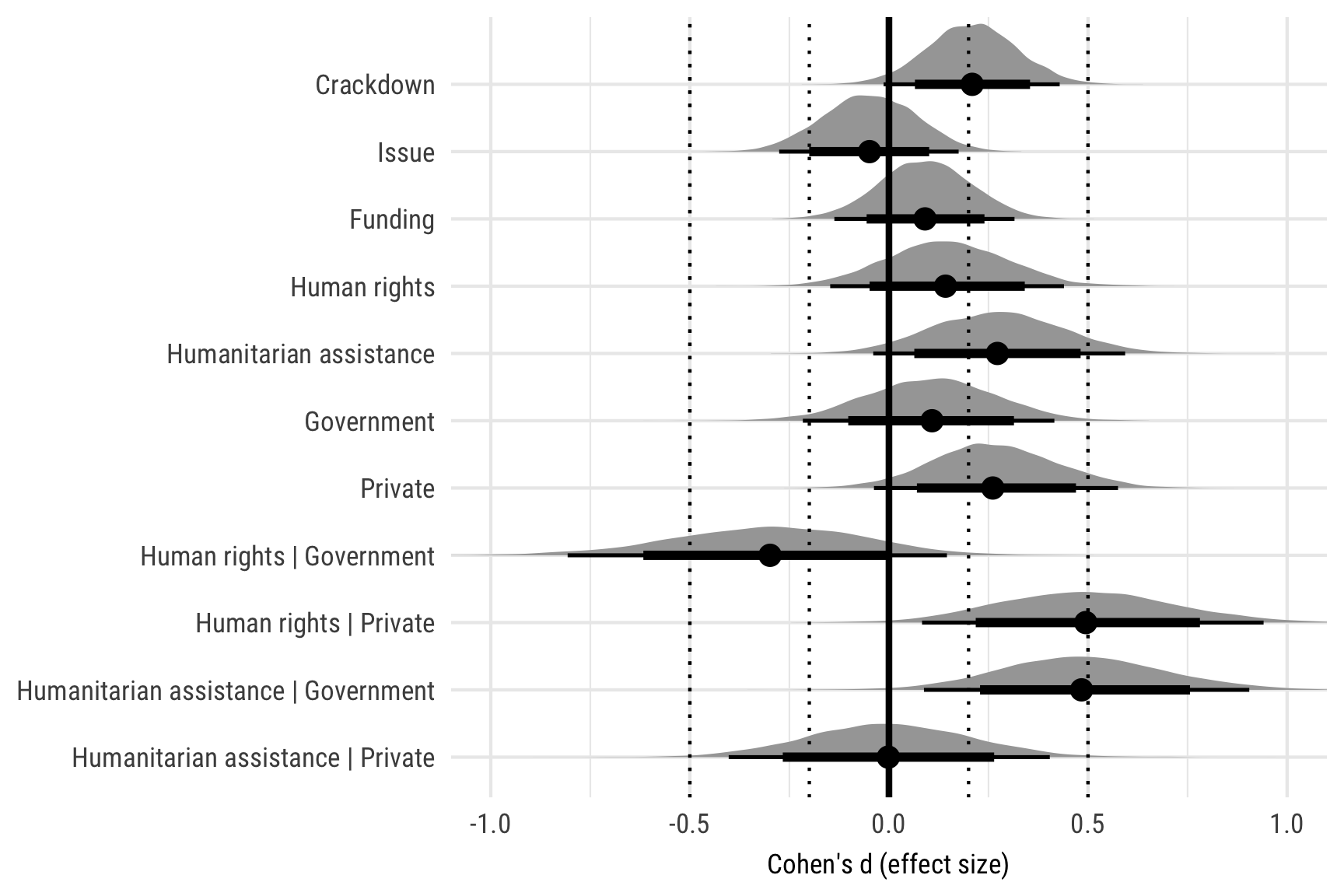Original computing environment

writeLines(readLines(file.path(Sys.getenv("HOME"), ".R/Makevars")))
## # http://dirk.eddelbuettel.com/blog/2017/11/27/#011_faster_package_installation_one
## VER=
## CCACHE=ccache
## CC=$(CCACHE) gcc$(VER)
## CXX=$(CCACHE) g++$(VER)
## CXXFLAGS=-O3 -Wno-unused-variable -Wno-unused-function -Wno-unused-local-typedefs
## CXX11=$(CCACHE) g++$(VER)
## CXX14=$(CCACHE) g++$(VER)
## FLIBS = -Lgfortran -print-file-name=libgfortran.dylib | xargs dirname
## FC=$(CCACHE) gfortran$(VER)
## F77=$(CCACHE) gfortran$(VER)
devtools::session_info()
## ─ Session info ──────────────────────────────────────────────────────────
##  setting  value
##  version  R version 3.5.2 (2018-12-20)
##  os       macOS Mojave 10.14.3
##  system   x86_64, darwin15.6.0
##  ui       X11
##  language (EN)
##  collate  en_US.UTF-8
##  ctype    en_US.UTF-8
##  tz       America/Denver
##  date     2019-03-13
##
## ─ Packages ──────────────────────────────────────────────────────────────
##  package                * version      date       lib
##  arrayhelpers             1.0-20160527 2016-05-28 
##  assertthat               0.2.0        2017-04-11 
##  backports                1.1.3        2018-12-14 
##  base64enc                0.1-3        2015-07-28 
##  bindr                    0.1.1        2018-03-13 
##  bindrcpp               * 0.2.2        2018-03-29 
##  boot                     1.3-20       2017-08-06 
##  broom                  * 0.5.1        2018-12-05 
##  callr                    3.1.1        2018-12-21 
##  cellranger               1.1.0        2016-07-27 
##  cli                      1.0.1        2018-09-25 
##  coda                     0.19-2       2018-10-08 
##  codetools                0.2-15       2016-10-05 
##  colorspace               1.4-0        2019-01-13 
##  crackdownsphilanthropy * 0.0.0.9000   2019-03-13 
##  crayon                   1.3.4        2017-09-16 
##  curl                     3.3          2019-01-10 
##  dagitty                  0.2-2        2016-08-26 
##  desc                     1.2.0        2018-05-01 
##  devtools                 2.0.1        2018-10-26 
##  digest                   0.6.18       2018-10-10 
##  dplyr                  * 0.7.8        2018-11-10 
##  evaluate                 0.13         2019-02-12 
##  farver                   1.1.0        2018-11-20 
##  forcats                * 0.3.0        2018-02-19 
##  fs                       1.2.6        2018-08-23 
##  generics                 0.0.2        2018-11-29 
##  ggdag                  * 0.1.0        2018-03-27 
##  ggforce                  0.1.3        2018-07-07 
##  ggplot2                * 3.1.0        2018-10-25 
##  ggraph                 * 1.0.2        2018-07-07 
##  ggrepel                  0.8.0        2018-05-09 
##  ggridges                 0.5.1        2018-09-27 
##  ggstance               * 0.3.1        2018-07-20 
##  glue                     1.3.0.9000   2019-02-09 
##  gridExtra                2.3          2017-09-09 
##  gtable                   0.2.0        2016-02-26 
##  haven                    2.0.0        2018-11-22 
##  here                   * 0.1          2017-05-28 
##  hms                      0.4.2        2018-03-10 
##  htmltools                0.3.6        2017-04-28 
##  httr                     1.4.0        2018-12-11 
##  igraph                   1.2.2        2018-07-27 
##  inline                   0.3.15       2018-05-18 
##  janitor                * 1.1.1        2018-07-31 
##  jsonlite                 1.6          2018-12-07 
##  knitr                    1.21         2018-12-10 
##  labeling                 0.3          2014-08-23 
##  lattice                  0.20-38      2018-11-04 
##  lazyeval                 0.2.1        2017-10-29 
##  loo                      2.0.0        2018-04-11 
##  lubridate                1.7.4        2018-04-11 
##  magrittr                 1.5          2014-11-22 
##  MASS                     7.3-51.1     2018-11-01 
##  matrixStats              0.54.0       2018-07-23 
##  memoise                  1.1.0        2017-04-21 
##  modelr                   0.1.2        2018-05-11 
##  munsell                  0.5.0        2018-06-12 
##  nlme                     3.1-137      2018-04-07 
##  pander                 * 0.6.3        2018-11-06 
##  patchwork              * 0.0.1        2019-01-24 
##  pillar                   1.3.1        2018-12-15 
##  pkgbuild                 1.0.2        2018-10-16 
##  pkgconfig                2.0.2        2018-08-16 
##  plyr                     1.8.4        2016-06-08 
##  prettyunits              1.0.2        2015-07-13 
##  processx                 3.2.1.9000   2019-03-07 
##  ps                       1.3.0        2018-12-21 
##  purrr                  * 0.3.1        2019-03-03 
##  R6                       2.4.0        2019-02-14 
##  Rcpp                   * 1.0.0        2018-11-07 
##  readr                  * 1.3.1        2018-12-21 
##  remotes                  2.0.2        2018-10-30 
##  rlang                    0.3.1        2019-01-08 
##  rmarkdown                1.11         2018-12-08 
##  rprojroot                1.3-2        2018-01-03 
##  rstan                  * 2.18.2       2018-11-07 
##  rstantools               1.5.1        2018-08-22 
##  rstudioapi               0.9.0        2019-01-09 
##  rvest                    0.3.2        2016-06-17 
##  scales                 * 1.0.0        2018-08-09 
##  sessioninfo              1.1.1        2018-11-05 
##  StanHeaders            * 2.18.1       2019-01-28 
##  stringi                  1.3.1        2019-02-13 
##  stringr                * 1.4.0        2019-02-10 
##  svUnit                   0.7-12       2014-03-02 
##  testthat                 2.0.1        2018-10-13 
##  tibble                 * 2.0.1        2019-01-12 
##  tidybayes              * 1.0.3        2018-10-22 
##  tidygraph                1.1.1        2018-11-20 
##  tidyr                  * 0.8.2        2018-10-28 
##  tidyselect               0.2.5        2018-10-11 
##  tidyverse              * 1.2.1        2017-11-14 
##  tweenr                   1.0.1        2018-12-14 
##  units                    0.6-2        2018-12-05 
##  usethis                  1.4.0        2018-08-14 
##  V8                       2.0          2019-02-07 
##  viridis                  0.5.1        2018-03-29 
##  viridisLite              0.3.0        2018-02-01 
##  withr                    2.1.2        2018-03-15 
##  xfun                     0.5          2019-02-20 
##  xml2                     1.2.0        2018-01-24 
##  yaml                     2.2.0        2018-07-25 
##  source
##  CRAN (R 3.5.0)
##  CRAN (R 3.5.0)
##  CRAN (R 3.5.0)
##  CRAN (R 3.5.0)
##  CRAN (R 3.5.0)
##  CRAN (R 3.5.0)
##  CRAN (R 3.5.2)
##  CRAN (R 3.5.0)
##  CRAN (R 3.5.0)
##  CRAN (R 3.5.0)
##  CRAN (R 3.5.0)
##  CRAN (R 3.5.0)
##  CRAN (R 3.5.2)
##  CRAN (R 3.5.2)
##  local
##  CRAN (R 3.5.0)
##  CRAN (R 3.5.2)
##  CRAN (R 3.5.0)
##  CRAN (R 3.5.0)
##  CRAN (R 3.5.2)
##  CRAN (R 3.5.0)
##  CRAN (R 3.5.0)
##  CRAN (R 3.5.2)
##  CRAN (R 3.5.0)
##  CRAN (R 3.5.0)
##  CRAN (R 3.5.0)
##  CRAN (R 3.5.0)
##  CRAN (R 3.5.0)
##  CRAN (R 3.5.0)
##  CRAN (R 3.5.0)
##  CRAN (R 3.5.0)
##  CRAN (R 3.5.0)
##  CRAN (R 3.5.0)
##  CRAN (R 3.5.0)
##  Github (tidyverse/glue@8188cea)
##  CRAN (R 3.5.0)
##  CRAN (R 3.5.0)
##  CRAN (R 3.5.0)
##  CRAN (R 3.5.0)
##  CRAN (R 3.5.0)
##  CRAN (R 3.5.0)
##  CRAN (R 3.5.0)
##  CRAN (R 3.5.0)
##  CRAN (R 3.5.0)
##  CRAN (R 3.5.0)
##  CRAN (R 3.5.0)
##  CRAN (R 3.5.2)
##  CRAN (R 3.5.0)
##  CRAN (R 3.5.2)
##  CRAN (R 3.5.0)
##  CRAN (R 3.5.0)
##  CRAN (R 3.5.0)
##  CRAN (R 3.5.0)
##  CRAN (R 3.5.2)
##  CRAN (R 3.5.0)
##  CRAN (R 3.5.0)
##  CRAN (R 3.5.0)
##  CRAN (R 3.5.0)
##  CRAN (R 3.5.2)
##  CRAN (R 3.5.0)
##  Github (thomasp85/patchwork@fd7958b)
##  CRAN (R 3.5.0)
##  CRAN (R 3.5.0)
##  CRAN (R 3.5.0)
##  CRAN (R 3.5.0)
##  CRAN (R 3.5.0)
##  CRAN (R 3.5.0)
##  Github (r-pkgs/processx@823819d)
##  CRAN (R 3.5.0)
##  CRAN (R 3.5.2)
##  CRAN (R 3.5.2)
##  CRAN (R 3.5.0)
##  CRAN (R 3.5.0)
##  CRAN (R 3.5.0)
##  CRAN (R 3.5.0)
##  CRAN (R 3.5.2)
##  CRAN (R 3.5.0)
##  CRAN (R 3.5.0)
##  CRAN (R 3.5.0)
##  CRAN (R 3.5.0)
##  CRAN (R 3.5.2)
##  CRAN (R 3.5.0)
##  CRAN (R 3.5.0)
##  CRAN (R 3.5.0)
##  CRAN (R 3.5.2)
##  CRAN (R 3.5.2)
##  CRAN (R 3.5.2)
##  CRAN (R 3.5.0)
##  CRAN (R 3.5.0)
##  CRAN (R 3.5.2)
##  CRAN (R 3.5.0)
##  CRAN (R 3.5.0)
##  CRAN (R 3.5.0)
##  CRAN (R 3.5.0)
##  CRAN (R 3.5.0)
##  CRAN (R 3.5.0)
##  CRAN (R 3.5.0)
##  CRAN (R 3.5.0)
##  CRAN (R 3.5.2)
##  CRAN (R 3.5.0)
##  CRAN (R 3.5.0)
##  CRAN (R 3.5.0)
##  CRAN (R 3.5.2)
##  CRAN (R 3.5.0)
##  CRAN (R 3.5.0)
##
##  /Library/Frameworks/R.framework/Versions/3.5/Resources/library